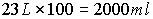Conversion Tables and Methods to Use when converting

from Metric Units to Metric Units (Volume)

Some terms to remember

 Meter – Length Kilo – Thousand Liter – Volume Milli – Thousand Gram – Mass/Weight Centi – Hundred Celsius – Temperature Deci – Ten

### Measure of Volumes

 10 milliliters (ml) = 1 centiliter (cl) 10 centiliters = 1 deciliter (dl) = 100 milliliters 1 liter = 1000 milliliters 1 milliliter = 1 cubic centimeter 1 liter = 1000 cubic centimeters 100 centiliter = 1 liter (L) = 1,000 milliliters 1000 liters = 1 kiloliter (km)

### Operations for Volume

Example: To get Milliliters from Liters you Multiply the Liters by 1000

You Have: 2 Liters

You want Cubic Centimeters: So, Cubic Centimeters = 23 Liters

We Multiply the liters by 100:You want Cubic Centimeters: So, Cubic Centimeters = 23 Liters There are 2000 milliliters and 2000 Cucbic Centimeters (CCs) in 2 liters

 If you have this Do this To get this milliliters (ml) or CCs Divide by 10 (ml/10) centiliter (cl) centiliters (cl) Multiply by 10 (cl * 10) Milliliters (ml) Liters (L) Multiply by 1000 (L * 100) Milliliters (mL) or CCs Liters (L) Multiply by 100 (L * 100) Centiliters (cl) Centiliters (cl) Divide by 100 (cl/100) Liters (L) Milliliters (ml) or CCs Divide by 1000 (ml/1000) Liters (L)

[page 1] [page 2] [page 3] [page 4] [page 5] [page 6] [page 7] [evaluation]

https://mste.illinois.edu/dildine/tcd_files/program15.htm